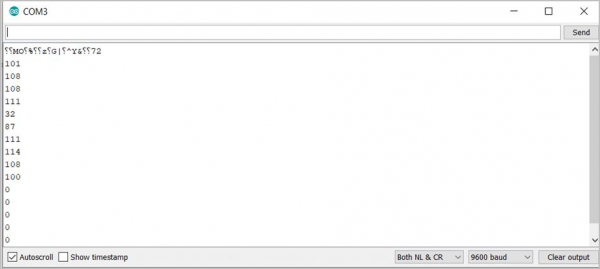# Arrays in Arduino

## Declaring an Array

In order to declare an array, you follow the syntax give below −

## Syntax

type array_name[array_size];

## Examples

char buf;
int new_array;

## Accessing elements of the array

The array element numbering starts from 0. The element can be accessed by specifying the index of the element in square brackets against the name of the array. For instance −

int second_element = new_array;

## Getting length of array

The length of the array can be accessed using the sizeof() function.

For example,

int buf_len = sizeof(buf);

Please note that the sizeof() function returns the number of bytes, and not the number of elements. If you have an int array, and int is represented as two bytes on your board, then this function will return double the length of your array. This can be avoided by the following:

int buf_len = sizeof(buf)/sizeof(buf);

## Populating an array

You can populate the array at the declaration stage itself. This will set all elements of the array to the value you set.

For example,

int new_array = 0;

will set all the 200 elements to 0.

For character array, you can also populate the array as follows −

char buf = "Hello World";

This will set the first element of the array to ‘H’, the next element to ‘e’, and so on. All the elements after “Hello World” will be set to 0. You can verify that by the following program −

## Example

char buf = "Hello World";
void setup() {
Serial.begin(9600);
}
void loop() {
// put your main code here, to run repeatedly:
for(int i = 0; i< sizeof(buf); i++){
Serial.println(buf[i], DEC);
delay(100);
}
}

## Output

The output of this program on the Serial Monitor is shown below −As you can see, all elements after ‘d’ (ASCII code 100) are 0. You can use the following ASCII table to verify the output: https://www.cs.cmu.edu/~pattis/15-1XX/common/handouts/ascii.html

Of course, individual elements of the array can also be populated separately, using the index. For example −

buf = 'a';

And so on.